# Algorithms¶

This part of the package provides a description, API and references to the implemented core algorithmic schemes (solvers) available in the SALSA package. Every algorithm can be supplied as a type to `salsa` subroutines either directly (see `salsa()`) or passed within `SALSAModel`. Please refer to Classification section for examples. Another available API is shipped with direct calls to algorithmic schemes. The latter is the most primitive and basic way of using SALSA package.

## Available high-level API¶

`PEGASOS`()

Defines an implementation (see `pegasos_alg()`) of the Pegasos: Primal Estimated sub-GrAdient SOlver for SVM which solves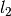-regularized problem defined here.

`L1RDA`()

Defines an implementation (see `l1rda_alg()`) of the l1-Regularized Dual Averaging solver which solves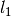-regularized problem defined here.

`ADA_L1RDA`()

Defines an implementation (see `adaptive_l1rda_alg()`) of the Adaptive l1-Regularized Dual Averaging solver which solves-regularized problem defined here in an adaptive way .

`R_L1RDA`()

Defines an implementation (see `reweighted_l1rda_alg()`) of the Reweighted l1-Regularized Dual Averaging solver which approximates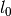-regularized problem in a limit.

`R_L2RDA`()

Defines an implementation (see `reweighted_l2rda_alg()`) of the Reweighted l2-Regularized Dual Averaging solver which approximates-regularized problem in a limit.

`SIMPLE_SGD`()

Defines an implementation (see `sgd_alg()`) of the unconstrained Stochastic Gradient Descent scheme which solves-regularized problem defined here.

`RK_MEANS`(support_alg, k_clusters, max_iter, metric)

Defines an implementation (see `stochastic_rk_means()`) of the Regularized Stochastic K-Means approach [JS2015]. Please refer to Clustering section for examples.

Parameters: support_alg – underlying support algorithm, e.g. `PEGASOS` k_clusters – number of clusters to be extracted max_iter – maximum number of outer iterations metric – metric to evaluate distances to centroids 

Selected `metric` unambiguously define a loss function used to learn centroids. Currently supported metrics are:

## Available low-level API¶

`pegasos_alg`(dfunc, X, Y, λ, k, max_iter, tolerance[, online_pass=0, train_idx=[]])
Parameters: dfunc – supplied loss function derivative (see `loss_derivative()`) X – training data (samples are stacked row-wise) represented by `Matrix`, `SparseMatrixCSC` or `DelimitedFile()` Y – training labels corresponding to `X` λ – trade-off hyperparameter k – sampling size at each iterationmax_iter – maximum number of iterations (budget) tolerance – early stopping threshold, i.e.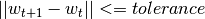online_pass – number of online passes through data, `online_pass=0` indicates a default stochastic mode instead of an online mode train_idx – subset of indices from `X` used to learn a model (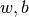)`sgd_alg`(dfunc, X, Y, λ, k, max_iter, tolerance[, online_pass=0, train_idx=[]])
Parameters: dfunc – supplied loss function derivative (see `loss_derivative()`) X – training data (samples are stacked row-wise) represented by `Matrix`, `SparseMatrixCSC` or `DelimitedFile()` Y – training labels corresponding to `X` λ – trade-off hyperparameter k – sampling size at each iterationmax_iter – maximum number of iterations (budget) tolerance – early stopping threshold, i.e.online_pass – number of online passes through data, `online_pass=0` indicates a default stochastic mode instead of an online mode train_idx – subset of indices from `X` used to learn a model ()`l1rda_alg`(dfunc, X, Y, λ, γ, ρ, k, max_iter, tolerance[, online_pass=0, train_idx=[]])
Parameters: dfunc – supplied loss function derivative (see `loss_derivative()`) X – training data (samples are stacked row-wise) represented by `Matrix`, `SparseMatrixCSC` or `DelimitedFile()` Y – training labels corresponding to `X` λ – trade-off hyperparameter γ – hyperparameter involved in elastic-net regularization ρ – hyperparameter involved in elastic-net regularization k – sampling size at each iterationmax_iter – maximum number of iterations (budget) tolerance – early stopping threshold, i.e.online_pass – number of online passes through data, `online_pass=0` indicates a default stochastic mode instead of an online mode train_idx – subset of indices from `X` used to learn a model ()`adaptive_l1rda_alg`(dfunc, X, Y, λ, γ, ρ, k, max_iter, tolerance[, online_pass=0, train_idx=[]])
Parameters: dfunc – supplied loss function derivative (see `loss_derivative()`) X – training data (samples are stacked row-wise) represented by `Matrix`, `SparseMatrixCSC` or `DelimitedFile()` Y – training labels corresponding to `X` λ – trade-off hyperparameter γ – hyperparameter involved in elastic-net regularization ρ – hyperparameter involved in elastic-net regularization k – sampling size at each iterationmax_iter – maximum number of iterations (budget) tolerance – early stopping threshold, i.e.online_pass – number of online passes through data, `online_pass=0` indicates a default stochastic mode instead of an online mode train_idx – subset of indices from `X` used to learn a model ()`reweighted_l1rda_alg`(dfunc, X, Y, λ, γ, ρ, ɛ, max_iter, tolerance[, online_pass=0, train_idx=[]])
Parameters: dfunc – supplied loss function derivative (see `loss_derivative()`) X – training data (samples are stacked row-wise) represented by `Matrix`, `SparseMatrixCSC` or `DelimitedFile()` Y – training labels corresponding to `X` λ – trade-off hyperparameter γ – hyperparameter involved in reweighted formulation of a regularization term ρ – hyperparameter involved in reweighted formulation of a regularization term ɛ – reweighting hyperparameter k – sampling size at each iterationmax_iter – maximum number of iterations (budget) tolerance – early stopping threshold, i.e.online_pass – number of online passes through data, `online_pass=0` indicates a default stochastic mode instead of an online mode train_idx – subset of indices from `X` used to learn a model ()`reweighted_l2rda_alg`(dfunc, X, Y, λ, ɛ, varɛ, max_iter, tolerance[, online_pass=0, train_idx=[]])
Parameters: dfunc – supplied loss function derivative (see `loss_derivative()`) X – training data (samples are stacked row-wise) represented by `Matrix`, `SparseMatrixCSC` or `DelimitedFile()` Y – training labels corresponding to `X` λ – trade-off hyperparameter ɛ – reweighting hyperparameter varɛ – sparsification hyperparameter k – sampling size at each iterationmax_iter – maximum number of iterations (budget) tolerance – early stopping threshold, i.e.online_pass – number of online passes through data, `online_pass=0` indicates a default stochastic mode instead of an online mode train_idx – subset of indices from `X` used to learn a model ()`stochastic_rk_means`(X, rk_means, alg_params, max_iter, tolerance[, online_pass=0, train_idx=[]])
Parameters: X – training data (samples are stacked row-wise) represented by `Matrix`, `SparseMatrixCSC` or `DelimitedFile()` rk_means – algorithm defined by `RK_MEANS()` alg_params – hyperparameter of the supporting algorithm in `rk_means.support_alg` k – sampling size at each iterationmax_iter – maximum number of iterations (budget) tolerance – early stopping threshold, i.e.online_pass – number of online passes through data, `online_pass=0` indicates a default stochastic mode instead of an online mode train_idx – subset of indices from `X` used to learn a model ()Footnotes

  adaptation is taken with respect to observed (sub)gradients of the loss function
  metric types are defined in Distances.jl package